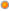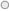Print

# Common Weight and Mass Conversions

User Rating: 3 / 5Enter figure :

Convert from:
To:
Result :

The weight converter calculates standard imperial units to metric and vice versa. It is also a useful tool for converting between common units, such as grams to kilograms or pounds to ounces.

To use the weight converter simply type in the desired amount you would like to covert then select which units you wish to convert from the drop down lists and click 'Convert'. The weight converter is particularly helpful for people wishing to know their body weight in either kilograms or pounds and converting weight measurements used in recipes.In making the calculations, the following conversions are used:

• 1 pound = 453.59237 grams
• 1 ounce = 0.0625 pound